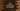# C programming example to check if two strings are anagram or not### C programming example to check if two strings are anagram or not:

In this tutorial, we will learn how to check if two strings are Anagram or not using C. Two strings are called anagram if letters of one string can be rearranged to create the other string. For example, cat and atc are anagram strings. Similarly, hello world and world hello are anagram. The user will provide both strings and our program will find out if they are anagram or not.

We will use ASCII values of characters to solve this problem. Let’s take a look at the algorithm :

### Algorithm :

• Our program is case sensitive . It will consider both Hello and hello as two different word.
• First initialize one integer array of size 128 with all element as 0.
• For each character of the first array, increment its corresponding position in this array by 1. For example, for character ‘a’, we will increment the value at position 97 since ASCII for a is 97.
• For the second string, decrement the value for each character position in the array similarly.
• So, if two strings are anagram, the array should contain 0 in all of its position.
• If any value is not zero, it is not anagram.
• We will ignore blank spaces while incrementing or decrementing the values.

For string strOne and strTwo, we may have strOne < strTwo or strOne > strTwo. For example :

``````"Hello World"
"H e l l o W o r l d"
``````

Both are anagram but length is different.

• Our program will scan both strings simultaneously. It will scan to the end of the first string. If the size of first string is 7, it will scan to the 7th character of both first and second string first.
• If the second string is smaller than the first,it will stop scanning the second string but keep scanning till the first string completes.
• If the second string is bigger than the first,after the first string is completed, it will keep scanning the second string.
• Each time,for the first string, it will increse its corresponding value and for the second string, it will decrease the value.
• For any space or balnk character, it will ignore that.

Let’s take a look at the program :

### C program to check Anagram strings :

``````#include <stdio.h>
#include <ctype.h>

void notifyAnagram(){
printf("Strings are anagram.\n");
}

void notifyNotAnagram(){
printf("Strings are not anagram.\n");
}

int main()
{
//1
int countArray = {0};
int position,i;
int isAnagram = 1;

//2
char firstString, secondString;

//3
printf("Enter the first string : ");
fgets(firstString,sizeof(firstString),stdin);

printf("Enter the second string : ");
fgets(secondString,sizeof(secondString),stdin);

position = 0;
int secondStringEnded = 0;

//4
while(firstString[position] != '\n'){
//5
if(!isspace(firstString[position])){
countArray[firstString[position]]++;
}

//6
if(secondString[position] == '\n'){
secondStringEnded = 1;
}

//7
if(secondStringEnded == 0 && !isspace(secondString[position])){
countArray[secondString[position]]--;
}

//8
position++;
}

//9
if(secondStringEnded == 0){
while(secondString[position] != '\n'){
if(!isspace(secondString[position])){
countArray[secondString[position]]--;
}
position ++;
}
}

//10
for(i=0; i < 128; i++){
if(countArray[i] != 0){
notifyNotAnagram();
isAnagram = 0;
break;
}
}

//11
if(isAnagram == 1){
notifyAnagram();
}

return 0;
}
``````

### Explanation :

The commented numbers in the above program denote the step number below :

1. Create countArray to store the count of ASCII value for each character.
2. Create two string array to store the strings.
3. Ask the user to enter both strings . Read and save it in firstString and secondString variables. secondStringEnded value determines if second string is completly scanned or not. 1 means yes.
4. Using one while loop scan each character of the firstString.
5. If the current character of firstString is not empty, increment the value for its ASCII position in the array.
6. If the second string is completed scanning, mark the flag as 1.
7. If the second string is not completed scanning and current value is not empty, decrement the value for its ASCII position in the array.
8. Increment the position to scan the next character position.
9. If the above loop is completed, means firstString is ended scanning. Now, if still few characters left in the secondString, scan them and decrement the count in the array.
10. At this point, both strings are scanned. Check each element of the countArray. If any element is not zero, print that the string is not anagram and mark flag isAnagram as 0.
11. If flag isAnagram is not zero, mark that the strings are anagram.

### Sample Output :

``````Enter the first string : helloworld
Enter the second string : hello world
Strings are anagram.

Enter the first string : hello world
Enter the second string : helloworld
Strings are anagram.

Enter the first string : he ll o w o r l d
Enter the second string : h l l e o r l d w o
Strings are anagram.

Enter the first string : rail safety
Enter the second string : fairy tales
Strings are anagram.
``````